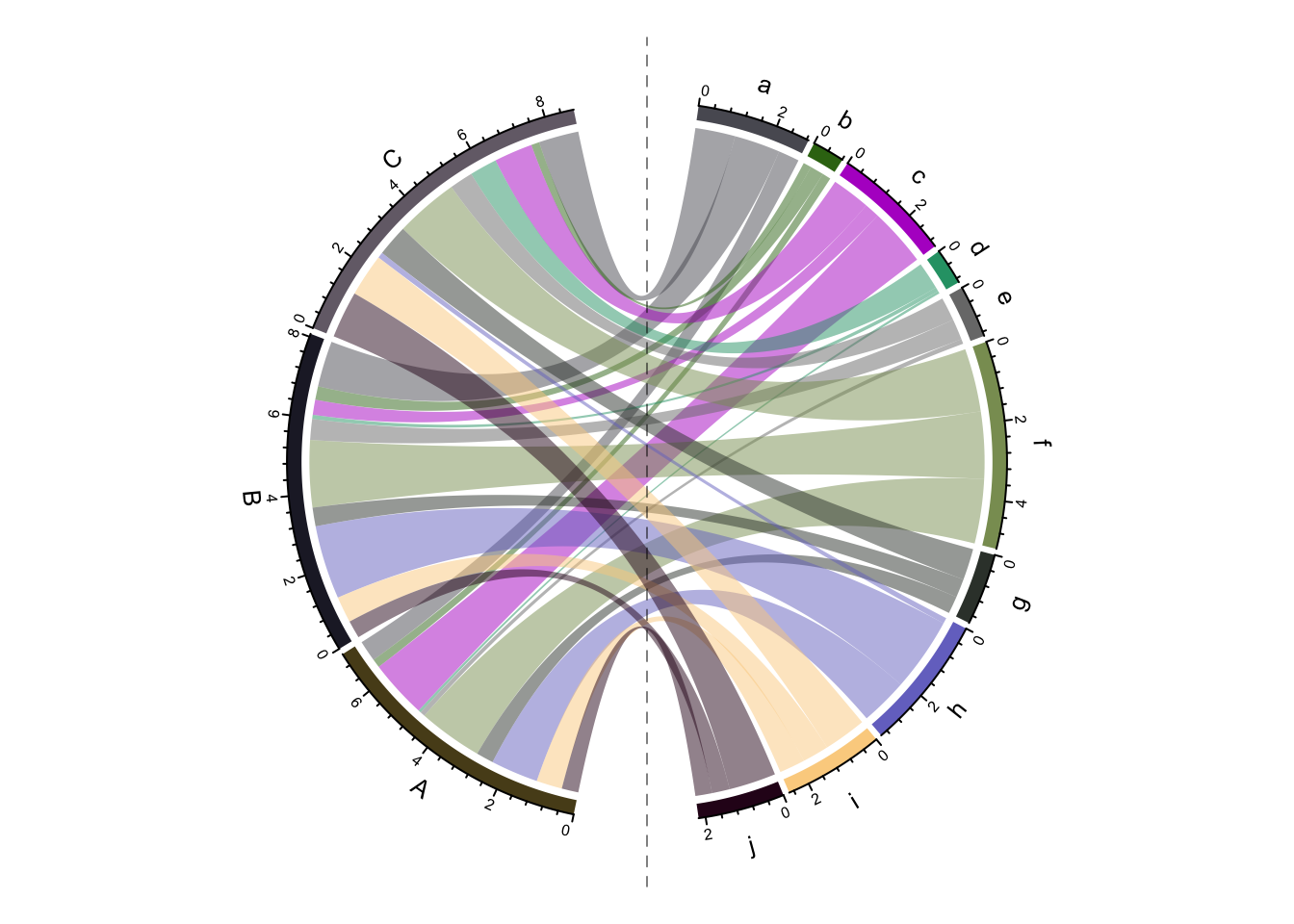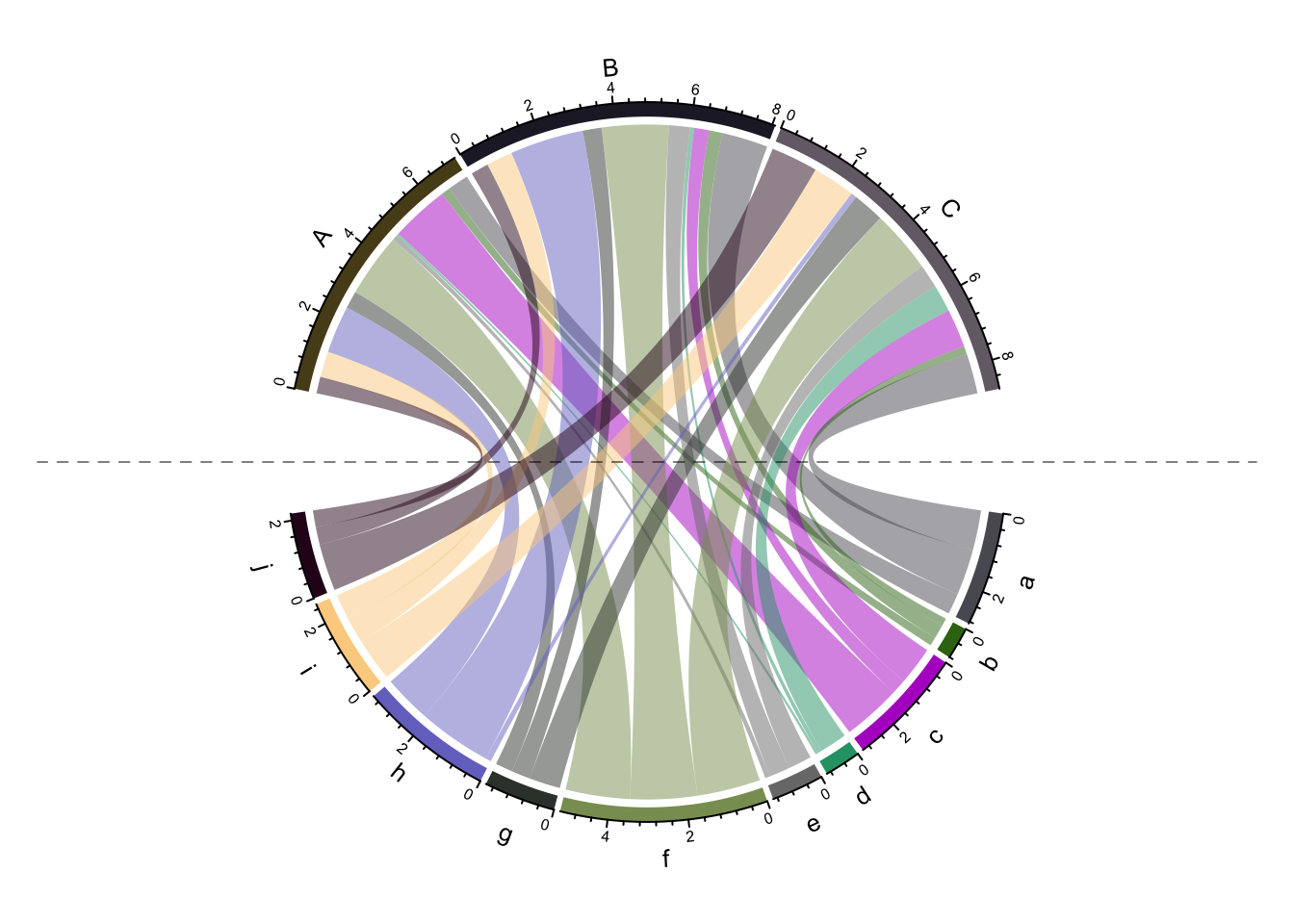# Put Chord diagram horizontally or vertically symmetric

In Chord diagram, when there are two groups (which correspond to rows and columns if the input is a adjacency matrix), it is always visually beautiful to rotate the diagram to be symmetric on horizontal direction or vertical direction. Actually it is quite easy to calculate a proper degree that needs to be rotated for the circle. In this post, I will show how to calculate such kind of “rotated degree value”.

First let’s create some random data.

``````set.seed(123)
mat = matrix(rnorm(30), nrow = 10, dimnames = list(letters[1:10], LETTERS[1:3]))
nr = nrow(mat)
nc = ncol(mat)
n_sector = nr + nc``````

In the Chord diagram, the total width of row sectors corresponds to the sum of row sum of the matrix with absolute values and so is for the column sectors.

``````row_sum = sum(rowSums(abs(mat)))
col_sum = sum(colSums(abs(mat)))``````

Small gaps between sectors are set to 1 degree and big gaps between row and column sectors are set to 20 degree.

``````small_gap = 1
big_gap = 20``````

In the circle, there are regions which are covered by small gaps, big gaps and sectors. Since the width of sectors are proportional to the row sums and/or column sums of the matrix, it is easy to calculate how much degrees are hold by the row sectors:

``````row_sector_degree = (360 - small_gap*(n_sector - 2) - big_gap*2) * (row_sum/(row_sum + col_sum)) +
small_gap*(nr-1)``````

If the row sectors are put in the right of the circle, we can calculate the “start degree” for the circle. Note `chordDiagram()` always draw row sectors first and by default the circle goes clockwisely.

``start_degree = 90 - (180 - row_sector_degree)/2``

Note there are small gaps and big gaps between sectors, the `gap.degree` in `circos.par()` should be set as a vector. I also added a vertical line which assists to see the symmetry.

``````gaps = c(rep(small_gap, nrow(mat) - 1), big_gap, rep(small_gap, ncol(mat) - 1), big_gap)
circos.par(gap.degree = gaps, start.degree = start_degree)
grid_col = structure(rand_color(n_sector), names = c(rownames(mat), colnames(mat)))
chordDiagram(mat, grid.col = grid_col)
circos.clear()
abline(v = 0, lty = 2, col = "#00000080")``````Similar we can adjust the “start degree” to let the circle looks horizonal.

``````start_degree = 0 - (180 - row_sector_degree)/2
gaps = c(rep(small_gap, nrow(mat) - 1), big_gap, rep(small_gap, ncol(mat) - 1), big_gap)
circos.par(gap.degree = gaps, start.degree = start_degree)
chordDiagram(mat, grid.col = grid_col)
circos.clear()
abline(h = 0, lty = 2, col = "#00000080")``````